# KVPY-SA 2019 Physics Paper with Solutions

KVPY-SA 2019 Paper with Solutions Physics is available here. Revise them and gain confidence, which in turn reduces the fear of facing the examination. It will help the students to attempt maximum questions in the stipulated time by increasing their speed and accuracy. Candidates can download the previous years’ question papers of KVPY to figure out the alternative and easy method to solve a particular problem. Practising the previous year papers is the best tool for the preparation of this competitive exam KVPY, in terms of the syllabus and distribution of marks across different topics. Solving these papers with solutions will boost the candidates’ confidence and scores in the KVPY examination.

### KVPY SA 2019 - Physics

Question 1: Various optical processes are involved in the formation of a rainbow. Which of the following provides the correct order in time in which these processes occur?

1. a. Refraction, total internal reflection, refraction
2. b. Total internal reflection, refraction, total internal reflection.
3. c. Total internal reflection, refraction, refraction.
4. d. Refraction, total internal reflection, total internal reflection.

Solution:

The rainbow result from refraction of sunlight in falling water droplets plus the reflection of the light from the back of the droplet.

Tiny droplets of water. Due to very less mass gets suspended in the atmosphere and acts as a good optical medium.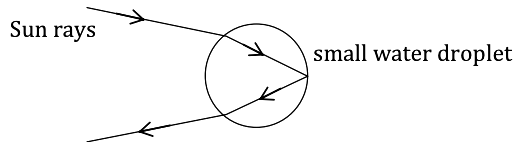When sunlight falls on small water droplet it suffers the first refraction, then total internal reflection and then refraction (as we can see in figure).

So, the correct order of the formation of a rainbow is refraction, total internal reflection and the refraction. So, the correct option is (a).

Question 2: A specially designed vernier calliper has the main scale least count of 1 mm. On the vernier scale, there are 10 equal divisions and they match with 11 main scale divisions. Then, the least count of the vernier calliper is

1. a. 0.1 mm
2. b. 0.909 mm
3. c. 1.1 mm
4. d. 0.09 mm

Solution:

Least count = 1 V.S.D. – 1 M.S.D.

Where V.S. D = Vernier scale division

M.S.D = Main scale division

Main scale division = 11

Vernier scale division = 10

Least count = 1 mm

So, the least count will be

Least count = [MSD / VSD] – 1

(L.C.) = [11 / 10] − 1 = [11 – 10] / 10 = 1 / 10 = 0.1mm

OR

Alternate solution

L.C. = (main scale least count)/(no. of the division of V.S.D which coincide with M.S.D.)

∴ L.C. = 1 / 10

∴ L.C. = 0.1 mm

Hence the correct option is (a).

Question 3: A steel ball is dropped in a viscous liquid. The distance of the steel ball from the top of the liquid is shown below. The terminal velocity of the ball is closest to.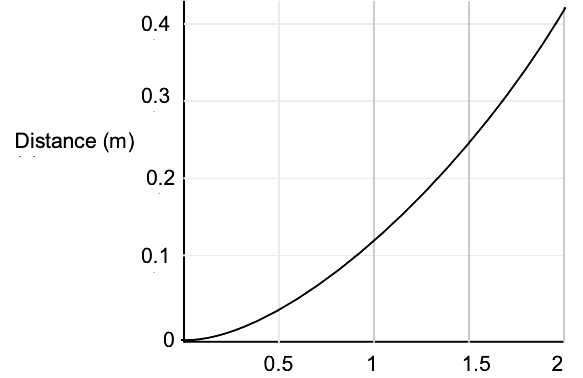1. a. 0.26 m/s
2. b. 0.33 m/s
3. c. 0.45 m/s
4. d. 0.21 m/s

Solution:

For any object when it reaches the terminal velocity, the graph should have a constant slope. In the given distance-time graph in the question, the slope of the graph is constant for the time1.6 second to 2 seconds.

When the time is 1.6s, the distance covered by the object is 0.3 m and when the time is 2s, the distance covered by the graph is 0.4 m.

So, the terminal velocity of the object found from the graph is

Vt = [x2 - x1] / [t2 - t1]

Here x2 = 0.4 m, x1 = 0.3

t2 = 1.9 sec, t1 = 1.6 sec

Vt = [0.4 - 0.3] / [1.9 - 1.6] = 0.1 / 0.3 = 0.33 m/s

So, the terminal velocity of the object is approximately 0.33 m/s.

Note: - Definite value of the distance is occurring from 1.6s to 1.9 s.

Hence, the correct option is (b).

Question 4: A student in a town in India, where the price per unit (1 unit = 1 kW-Hr) of electricity is Rs. 5.00. Purchases a 1 kVA UPS (uninterrupted power supply) battery. A day before the exam, 10 friends arrive at the student's home with their laptops and all connect their laptops to the UPS. Assume that each laptop has a constant power requirement of 90 W. Consider the following statements.

I .All the 10 laptops can be powered by the UPS if connected directly.

II. All the 10 laptops can be powered if connected using an extension box with a 3 A fuse.

III. If all the 10 friends use the laptop for 5 hours, then the cost of the consumed electricity is about Rs.22.50.

Select the correct option with true statements.

1. a. I only
2. b. I and II only
3. c. I and III only
4. d. II and III only

Solution:

UPS battery is of 1 KVA so power delivered by the battery will be 1000 W.

For each laptop power required is 90W.

∴ For 10 laptop total power requirement = 90 × 10 = 900 W

As we can see that the delivered power by the battery is greater than the required power. So, the statement I is correct.

As we know that power = P = VI

Where V = voltage, I = current

In India voltage used is 220 V

∴ I = P / V = 900 / 220 = 4.1A

So, statement II is incorrect.

Now if all the laptops are used for 5 hours then the consumed unit = [900 * 5 * 3600] / 3600000 = 4.5

Total pay amount or total cost of consumed electricity in 5 hours is = 4.5 unit × 5 = 22.5 rupees.

Hence the correct option is (c).

Question 5: Frosted glass is widely used for translucent windows. The region where a transparent adhesive tape is stuck over the frosted glass becomes transparent. The most reasonable explanation for this is

1. a. Diffusion of adhesive glue into the glass
2. b. Chemical reaction at the adhesive tape - glass interface.
3. c. Refractive index of adhesive glue is close to that of glass
4. d. Adhesive tape is more transparent than glass.

Solution:

Frosted glass has a different refractive index on its surface as frosted glass is produced by coating on the surface. Now as a transparent adhesive tape is stuck in the glass surface, the refractive index of the upper coating is adjusted to be same as that of glass, so it becomes transparent now.

If the refractive index of adhesive glue is different from that of glass then there is a chance of total internal reflection. Then the adhesive tape will not be transparent. Hence the correct option is (c).

Question 6: Consider two equivalent, triangular hollow prisms A and B made of thin glass plates and arranged with negligible spacing as shown in the figure. A beam of white light is incident on prism A from the left. Given that the refractive index of water is inversely related to temperature, the beam to the right of prism B would NOT appear white if –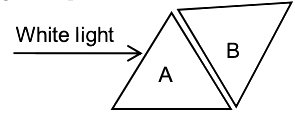1. a. both prisms are filled with hot water (70°C)
2. b. both prisms are filled with cold water (7°C)
3. c. both prisms are empty
4. d. prism A is filled with hot water (70°C) and prism B with cold water (7°C)

Solution:

The white light will appear as white light after passing through prisms only if the refractive index of both prism parts is the same; Since the effects of dispersion are cancelled out. Since the refractive index of both parts will not be the same when parts are filled with water at different temperatures.

Hence white light will not come out as white light.

Hence the correct option is (d).

Question 7: A ball is moving uniformly in a circular path of radius 1 m with a time period of 1.5 s. If the ball is suddenly stopped at t = 8.3 s, the magnitude of the displacement of the ball with respect to its position at t = 0 s is closest to -

1. a. 1 m
2. b. 33 m
3. c. 3 m
4. d. 2m

Solution:

As we know that the time period is given by T = 2𝛑 / 𝛚 = 1.5 = (where 𝛚 is angular velocity)

𝛚 = 2𝛑 / 1.5 ……………. (1)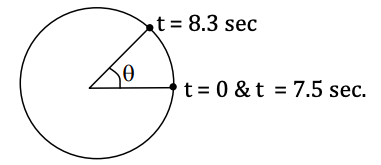Since the time period is 1.5 sec, the ball takes 5 full circles in 7.5 seconds.

The ball is stopped at t = 8.3 sec.

Remaining time = 8.3 – 7.5

= 0.8 sec.

∴ Angular displacement θ = 𝛚t

Substituting the value of 𝛚 from equation (1)

θ = [2𝛑 / 1.5] × 0.8

≅ 3.14

∴ Displacement ≅ 2R

≅ 2m.

Hence the correct option is (d).

Question 8: A particle slides from the top of a smooth hemispherical surface of radius R which is fixed on a horizontal surface. If it separates from the hemisphere at a height h from the horizontal surface then the speed of the particle is -

1. a. √(2g (R – h))
2. b. √(2g (R + h))
3. c. √(2gR)
4. d. √(2gh)

Solution: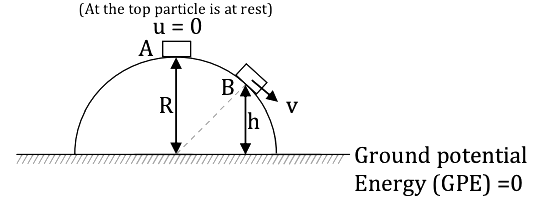Let the mass of the block is m and the block leaves contact at point B, which is on hemisphere at a height 'h' from the ground.

∴ By using conservation of energy between points A and B.

mgR + (1 / 2) mu2 = mgh + (1 / 2) mv2

m = mass of block

u = initial velocity (at point A)

h = height of block from ground at point B

v = final velocity (at point B)

mgR + 0 = mgh + (1 / 2) mv2

(1 / 2) mv2 = mg (R – h) (or by work energy theorem)

V2 = 2g (R – h)

V = √(2g(R − h))

Hence the correct option is (a).

Question 9: The nuclear radius is given by R = r0A1/3, where r0 is constant and A is the atomic mass number. Then -

1. a. The nuclear mass density of U238 is twice that of Sn119
2. b. The nuclear mass density of U238 is thrice that of Sn119
3. c. The nuclear mass density of U238 is the same as that of Sn119
4. d. The nuclear mass density of U238 is half that of Sn119

Solution:

Density of nuclear matter (D) = Mass / Volume

= [A. mp] / [(4 / 3) 𝛑R3] ……………. (1)

mp → mass of a proton

A → atomic mass number

Given; R = r0A1/3,

Substituting the value of R in equation (1)

D = [A. mp] / [(4 / 3) 𝛑{r0A1/3}3]

D = 3mp / 4𝛑r03 = constant

Mass density does not depend on mass number (A) and atomic number (Z).

Hence the correct option is (c).

Question 10: The electrostatic energy of a nucleus of charge Ze is equal to kZ2e 2 / R, where k is a constant and R is the nuclear radius. The nucleus divides into two daughter nuclei of charges Ze / 2 and equal radii. The change in electrostatic energy in the process when they are far apart is -

1. a. 0.375 kZ2e2 / R
2. b. 0.125 kZ2e2 / R
3. c. kZ2e2 / R
4. d. 0.5 kZ2e2 / R

Solution:

Initial electrostatic energy of nucleus:

Ei = Kz2e2 / R

K = constant, Ze = Charge of nucleus, R = Nuclear radius.

Now it breaks into two nuclei with radii R0 each.

So, by volume conservation.

Volume of the nucleus (initially) = volume of daughter nuclei

(4 / 3) 𝛑R3 = 2 * [(4 / 3) 𝛑R03]

R0 = R / (2)

Now, both nuclei separate to the large distance,

So, total energy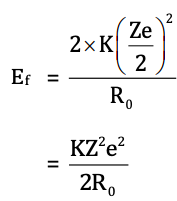Substituting the value of R0

= [KZ2e2 / 2R] 

= [0.63 KZ2e2] / R

Hence the change in energy

Ef - Ei = [KZ2e2 / R] [1– 0.67]

Ef - Ei = [0.37] [KZ2e2 / R]

Hence the correct option is (a).

Question 11: Two masses M1 and M2 carry positive charges Q1 and Q2, respectively. They are dropped to the floor in a laboratory setup from the same height where there is a constant electric field vertically upwards. M1 hits the floor before M2. Then -

1. a. Q1 > Q2
2. b. Q1 > Q2
3. c. M1Q1 > M2Q2
4. d. M1Q2 > M2Q1

Solution:

Given that,

M1 hits the floor before M2 it is possible when the acceleration of the mass M1 is greater than the acceleration of mass M2.

i.e. aM1 > aM2 ………… (1)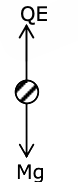In equilibrium Fnet = ma

Mg – QE = Ma

Fe = QE [Electrostatic force]

a = [Mg - QE] / M

For mass M1

a1 = [M1g - Q1E] / M1

For mass M2

a2 = [M2g - Q2E] / M2

Substituting the value aM1 and aM2 in equation (1) we get

[M1g - Q1E] / M1 > [M2g - Q2E] / M2

g - (Q1E / M1) > g - (Q2E / M2)

- (Q1E / M1) > - (Q2E / M2)

(Q1E / M1) < (Q2E / M2)

M1Q2 > M2Q1

Hence the correct option is (d).

Question 12: Which one of the following schematic graphs best represents the variation of PV (in Joules) versus T (in Kelvin) of one mole of an ideal gas? (The dotted line represents PV = T).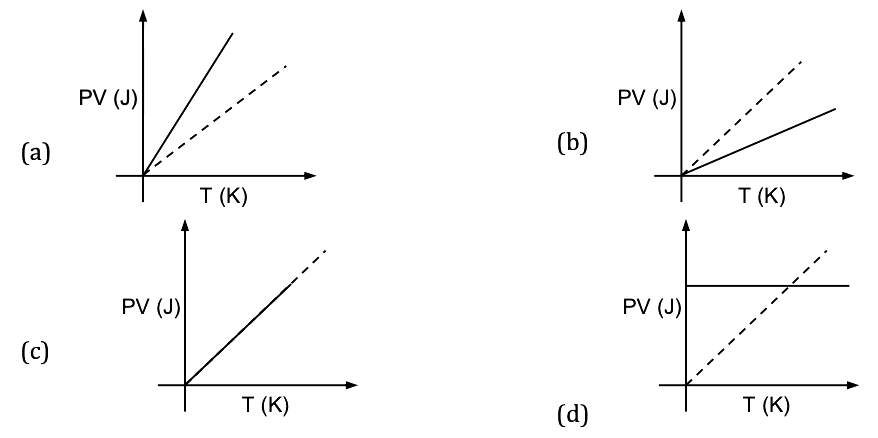Solution:

From ideal gas equation

PV = nRT

P = Pressure, V = Volume, n = No of moles, R = universal gas constant = 8.314 JK–1mol–1, T = temperature.

For n = 1

PV = RT

Here, R is the slope of PVvs T graph

PV = 8.314 T

Now, when compared to PV = T {Represented by dotted lines}

PV = 8.314 T is having more slope than PV = T.

Hence the correct option is (a).

Question 13: Mumbai needs 1.4 × 1012 litres of water annually. Its effective surface area is 600 km2 and it receives an average rainfall of 2.4 m annually. If 10% of this rainwater is conserved it will meet approximately -

1. a. 1% of Mumbai’s water needs
2. b. 10% of Mumbai’s water needs
3. c. 50% of Mumbai’s water needs
4. d. 100% of Mumbai’s water needs

Solution:

Mumbai's area = 600 Km2

= 600 × 106 m2

= 6 × 108 m2

Height of annual rainfall received: h = 2.4 m

∴ Water received by rain = 6 × 108 × 2.4 = 1.4 × 109 m3

10% of rain water is conserved therefore,

Conserved water = 1.4 × 109 × [10 / 100]

= 1.4 × 108 m3

Water needed annually = 1.4 × 1012 litres

= 1.4 × 109 m3

∴ Percentage of water received = {[1.4 × 108] / [1.4 × 109]} * 100

= 10%

Hence the correct option is (b).

Question 14: A mass M moving with a certain speed V collides elastically with another stationary mass m. After the collision, the masses M and m move with speeds V and respectively. All motion is in one dimension. Then-

1. a. V = V’ + v
2. b. V’ = V + v
3. c. V’ = (V + v) / 2
4. d. v = V + V’

Solution: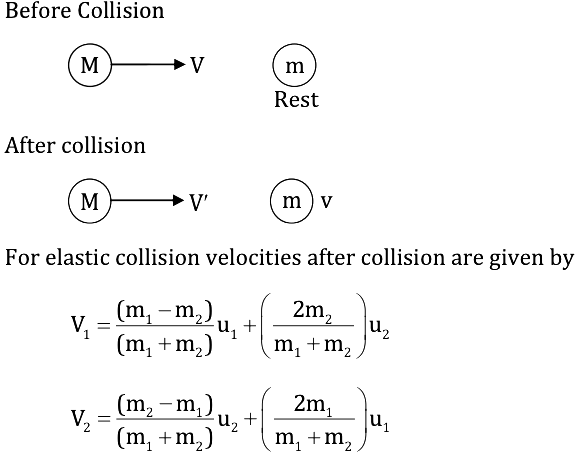Now using;

u1 = V, u2 = 0

V1 = V’, V2 = v

m1 = M, m2 = m

V1 = V’ = {[M – m] / [M + m]}V …… (1)

V2 = v = {[2M] / [M + m]}V …… (2)

Subtracting equation (1) and (2)

V’ - v = [(M – m) – 2M]V / (M + m)

V' – v = - V

V' + V = v

Hence the correct option is (d).

Question 15: Four rays, 1, 2, 3 and 4 are incident normally on the face PQ of an isosceles prism PQR with apex angle ∠Q = 120°. The refractive indices of the material of the prism for the above rays 1, 2, 3 and 4 are 1.85, 1.95, 2.05 and 2.15, respectively and the surrounding medium is air. Then the rays emerging from the face QR are-

1. a. 4 only
2. b. 1 and 2 only
3. c. 3 and 4 only
4. d. 1, 2, 3 and 4

Solution: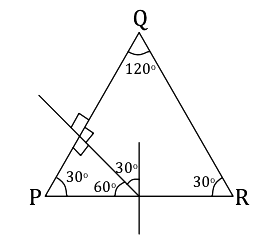For apex angle 120o and normal incidence on PQ. For a ray to pass through QR it will get totally internally reflected on PR. This means ray will not directly go to QR as shown in the figure.

For all ray’s angle of incidence on PR is 30o.

For TIR at PR

sin-1 (1 / μ) < 30o

(1 / μ) < (1 / 2)

μ > 2

According the question μ1 < μ2 < μ3 < μ4,

Here only for 3 and 4μ is greater than 2.

So, ray 3 and4 will emerge out.

Hence the correct option is (c).

Question 16: A water-proof laser pointer of length 10 cm placed in a water tank rotates about a horizontal axis passing through its centre of mass in a vertical plane as shown in the figure. The time period of rotation is the 60s. Assuming the water to be still and no reflections from the surface of the tank, the duration for which the light beam escapes the tank in one time period is close to (Refractive index of water = 1.33)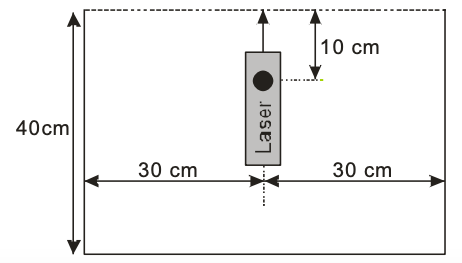1. a. 8.13 s
2. b. 14.05 s
3. c. 16.27 s
4. d. 23.86 s

Solution: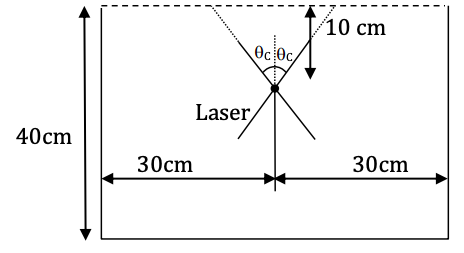Light will come out until the critical angle (θC),

μ1sin θC = μ2sin 90o

(4 / 3) sin θC = 1 * 1

sin θC = 3 / 4

θC ≅ 50o

θC = [50 * 𝛑] /  rad

For 𝛚

C = 𝛚t [𝛚 = θ / t, here θ = 2θC]

t = 2θC / 𝛚

= [2 * 50 * 𝛑 * 60] / [2𝛑 * 180]

t ≅ 16.27sec.

Hence the correct option is (c).

Question 17: In an hour-glass approximately 100 grains of sand fall per second (starting from rest), and it takes 2 sec for each sand particle to reach the bottom of the hour-glass. If the average mass of each sand particle is 0.2 g then the average force exerted by the falling sand on the bottom of the hour-glass is close to

1. a. 0.4 N
2. b. 0.8 N
3. c. 1.2 N
4. d. 1.6N

Solution:

The velocity of sand particle just before striking the bottom.

V = u + at (1st equation of motion)

V = 0 + 10 × 2 [u = 0 and t = 2s given]

V = 20 m/s

For 1 grain pi (Momentum just before the collision at the bottom) = mVi

pi = (0.2 × 10-3) × 20

= 4 × 10-3

pf (momentum after collision at bottom) = mVf

pf = (0.2 × 10-3) × 0

pf = 0 [ Final velocity = 0]

Change in momentum

|Δp| = |pf - pi|

= |0 - 4 * 10-3|

= 4 * 10-3 Kg m/s

Rate of change of momentum [|Δp| / [Δt]] = force exerted by falling sand

Force exert by 100 grains in 1 sec (faverage) = {[Δp] / [Δt]}* 100

= {[4 * 10-3] / 1} * 100

= 0.4 N

Hence the correct option is (a).

Question 18: A student uses the resistance of a known resistor (1 Ω) to calibrate a voltmeter and an ammeter using the circuits show below. The student measures the ratio of the voltage to current to be 1 × 103 Ω in-circuit (a) and 0.999 Ω in the circuit (b). From these measurements, the resistances (in Ω) of the voltmeter and ammeter are found to be close to: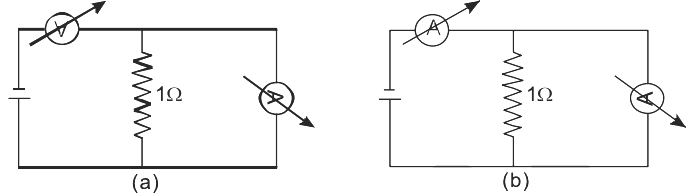1. a. 102 and 10–2
2. b. 103 and 10–3
3. c. 10–2 and 102
4. d. 10–3 and 103

Solution:

Case - (a)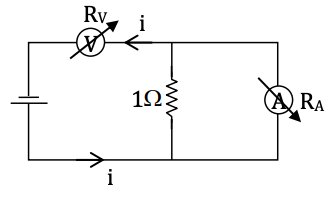Let, RV → resistance of the voltmeter

RA → resistance of the ammeter

The ratio of voltage to current (V / A) = i (RV) / [i / 1 + RA] = RV (1 + RA)

RV (1 + RA) = 1000 …… (1)

Case – (b)The ratio of voltage to current

(V / A) = {[i / 1 + RV] * RV} / i = RV / [RV + 1] = 0.999 …… (2)

RV = 0.999 + 0.999 RV

Resistance of voltmeter; RV = 999 V

RV ≅ 103 V

Putting this value of Rv in equation (1)

103 (1 + RA) = 1000

103 R = 1

RA = 10-3 Ω

Hence the correct option is (b).

Question 19: A hot air balloon with a payload rises in the air. Assume that the balloon is spherical in shape with a diameter of 11.7 m and the mass of the balloon and the payload (without the hot air inside) is 210 kg. Temperature and pressure of outside air are 27 °C and 1 atm = 105 N/m2 respectively. The molar mass of dry air is 30 g. The temperature of the hot air inside is close to, [The gas constant R = 8.31 J/K/mol]

1. a. 27°C
2. b. 52°C
3. c. 105°C
4. d. 171°C

Solution:

Here, the mass of the balloon and payload, m = 210 kg

Diameter of the balloon, d = 2r = 11.7 m

Air pressure (outside), P = 105 N/m2

Temperature of outside air, T0 = 27°C = 300k

𝛒in = density of air inside balloon

𝛒0 = density of air outside balloon

210 g + V𝛒ing = V𝛒0g

V (𝛒0 - 𝛒in) g = 210g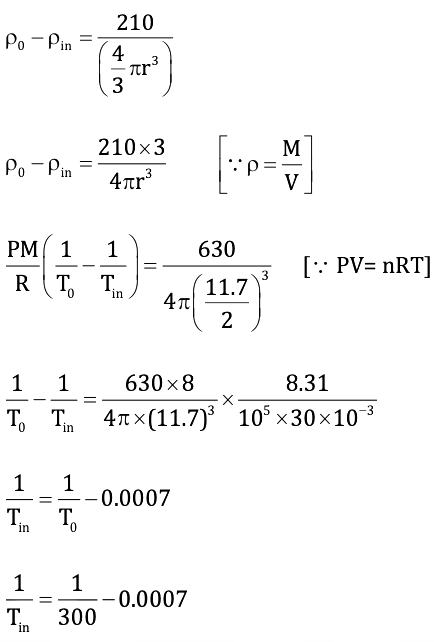Tin = 380.22 K.

Tin = 107.22oC ≅ 105oC

Hence the correct option is (c).

Question 20: A healthy adult of height 1.7 m has an average blood pressure (BP) of 100 mm of Hg. The heart is typically at a height of 1.3 m from the foot. Take the density of blood to be 103 kg/m3 and note that 100 mm of Hg is equivalent to 13.3 kPa (kilo Pascals). The ratio of BP in the foot region to that in the head region is close to

1. a. one
2. b. two
3. c. three
4. d. four

Solution: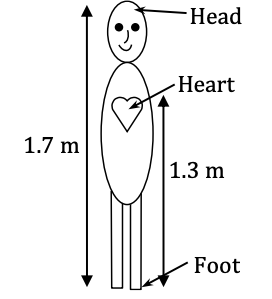Height from heart to feet = 1.3 m

Density of blood = 103 kg/m3

Pressure at the heart level

= 100 mm of Hg = 13.3 kPa

Total pressure of the feet of man

PFoot = PHeart + 𝛒gh

= 13.3 + 103 × 10 × 1.3 = 26.3 kPa

Phead = Pheart – 𝛒gh= 13.3 – 103 × 10 × (1.7 – 1.3)

= 9.3 kPa

PFoot / PHead = 26.3 / 9.3 = 3

Hence the correct option is (c).

### KVPY SA 2019 Physics Paper with Solutions